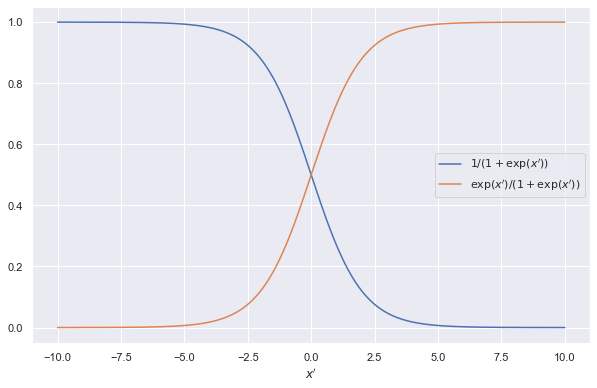In a classification problem, given a list of features values $x$ and their corresponding classes $\{c_i\}$, the posterior for of the classes, aka conditional probability of the classes, is

$$p(C=c_i\mid X=x).$$

Likelihood

The likelihood of the data is

$$p(X=x\mid C=c_i).$$

## Logistic Regression for Two Classes

For two classes, the simplest model for the posterior is a linear model,

$$\log \frac{p(C=c_1\mid X=x) }{p(C=c_2\mid X=x)} = \beta_0 + \beta_1 \cdot x,$$

which is equivalent to

$$p(C=c_1\mid X=x) = \exp\left(\beta_0 + \beta_1 \cdot x\right) p(C=c_2\mid X=x) .$$

Why

The reason that we proposing a linear model for the quantity

$$\log \frac{p(C=c_1\mid X=x) }{p(C=c_2\mid X=x)},$$

is that it has a range from $-\infty$ to $\infty$ which matches the range of the linear model $\beta_0 + \beta_1 \cdot x$.

We can also see in the following results that such relation guarantees that the conditional probabilities are restricted to 0 to 1 after applying the normalization constraint.

Using the normalization condition

$$p(C=c_1\mid X=x) + p(C=c_2\mid X=x) = 1,$$

we can derive the posterior for each classes

\begin{align} p(C=c_2\mid X=x) &= \frac{1}{1 + \exp\left(\beta_0 + \beta_1 \cdot x\right)} \\ p(C=c_1\mid X=x) &= \frac{\exp\left(\beta_0 + \beta_1 \cdot x\right)}{1 + \exp\left(\beta_0 + \beta_1 \cdot x\right)}. \end{align}The two conditional probabilitiesFor simplicity, we are using $x’=\beta_0 + \beta_1 \cdot x$ in this figure.

This is the

Limiting behavior

1. As $\beta_0 + \beta_1 \cdot x \to \infty$, we have $p(C=c_2\mid X=x) \to 0$ and $p(C=c_1\mid X=x)\to 1$.
2. As $\beta_0 + \beta_1 \cdot x \to 0$, we have $p(C=c_2\mid X=x) \to 0.5$ and $p(C=c_1\mid X=x)\to 0.5$.
3. As $\beta_0 + \beta_1 \cdot x \to -\infty$, we have $p(C=c_2\mid X=x) \to 1$ and $p(C=c_1\mid X=x)\to 0$.

## Relation to Cross Entropy

For two classes, we can write down the likelihood as

$$\pi_{i=1}^{N} p^{y_i} p^{1-y_i},$$

where $p$ is the probability of label $y_i=c_1$ and $1-p$ is probability of label $y_i=c_2$.

Taking the neglog, we find that

$$-l = sum_{i=1}^N ( -y_i \log p - (1-y_i)\log (1-p) ).$$

This is the

## Logistic Regression for $K$ Classes

It is easily generalized to problems with $K$ classes.

\begin{align} p(C=c_K\mid X=x) &= \frac{1}{1 + \sum_k\exp\left(\beta_{k0} + \beta_k \cdot x\right)} \\ p(C=c_k\mid X=x) &= \frac{\exp\left(\beta_{k0} + \beta_k \cdot x\right)}{1 + \sum_k\exp\left(\beta_{k0} + \beta_k \cdot x\right)} \end{align}

## Why not non-linear

The log of the posterior ratio can be more complex than linear models. In general, we have1

$$\log \frac{p(C=c_1\mid X=x) }{p(C=c_2\mid X=x)} = f(x),$$

so that

$$p(C=c_1\mid X=x) = \frac{\exp(f(x))}{ 1 + \exp(f(x)) }.$$

The logistic regression model we mentioned in the previous sections require

$$f(x) = \beta_0 + \beta_1 \cdot x.$$

A more general additive model is

$$f(x) = \sum_i f_i(x),$$

where we can apply algorithms such as local scoring to fit such models1.

Planted: by ;

L Ma (2021). 'Logistic Regression', Datumorphism, 05 April. Available at: https://datumorphism.leima.is/wiki/machine-learning/linear/logistic-regression/.